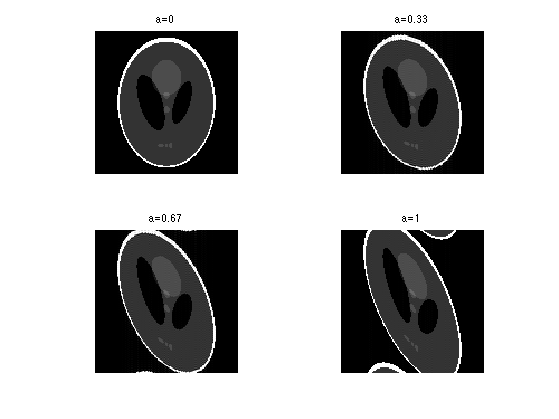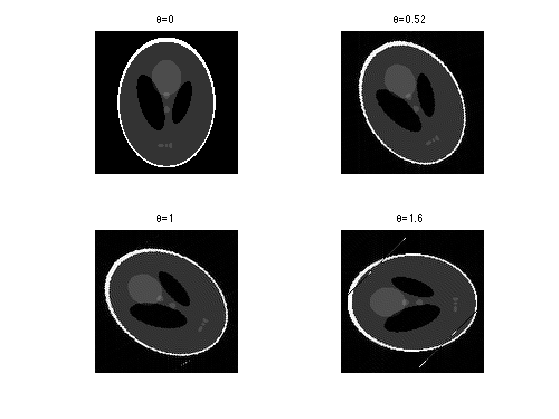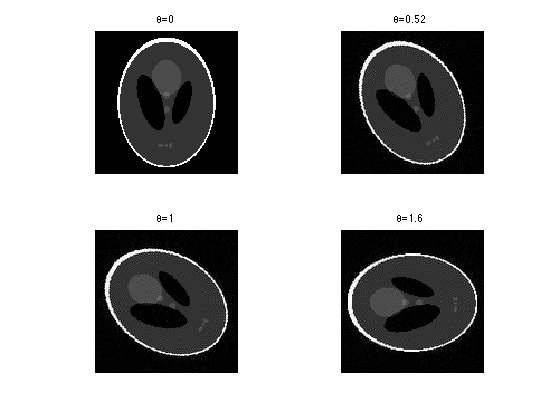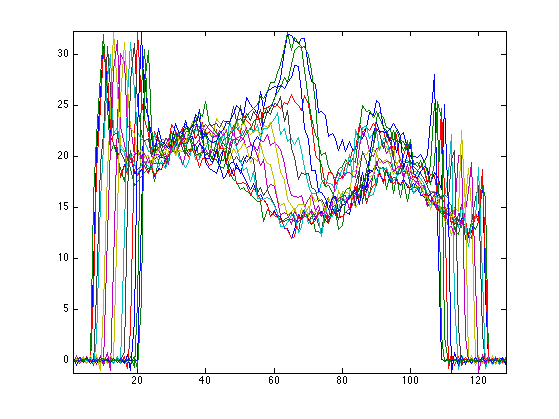$\newcommand{\NN}{\mathbb{N}} \newcommand{\CC}{\mathbb{C}} \newcommand{\GG}{\mathbb{G}} \newcommand{\LL}{\mathbb{L}} \newcommand{\PP}{\mathbb{P}} \newcommand{\QQ}{\mathbb{Q}} \newcommand{\RR}{\mathbb{R}} \newcommand{\VV}{\mathbb{V}} \newcommand{\ZZ}{\mathbb{Z}} \newcommand{\FF}{\mathbb{F}} \newcommand{\KK}{\mathbb{K}} \newcommand{\UU}{\mathbb{U}} \newcommand{\EE}{\mathbb{E}} \newcommand{\Aa}{\mathcal{A}} \newcommand{\Bb}{\mathcal{B}} \newcommand{\Cc}{\mathcal{C}} \newcommand{\Dd}{\mathcal{D}} \newcommand{\Ee}{\mathcal{E}} \newcommand{\Ff}{\mathcal{F}} \newcommand{\Gg}{\mathcal{G}} \newcommand{\Hh}{\mathcal{H}} \newcommand{\Ii}{\mathcal{I}} \newcommand{\Jj}{\mathcal{J}} \newcommand{\Kk}{\mathcal{K}} \newcommand{\Ll}{\mathcal{L}} \newcommand{\Mm}{\mathcal{M}} \newcommand{\Nn}{\mathcal{N}} \newcommand{\Oo}{\mathcal{O}} \newcommand{\Pp}{\mathcal{P}} \newcommand{\Qq}{\mathcal{Q}} \newcommand{\Rr}{\mathcal{R}} \newcommand{\Ss}{\mathcal{S}} \newcommand{\Tt}{\mathcal{T}} \newcommand{\Uu}{\mathcal{U}} \newcommand{\Vv}{\mathcal{V}} \newcommand{\Ww}{\mathcal{W}} \newcommand{\Xx}{\mathcal{X}} \newcommand{\Yy}{\mathcal{Y}} \newcommand{\Zz}{\mathcal{Z}} \newcommand{\al}{\alpha} \newcommand{\la}{\lambda} \newcommand{\ga}{\gamma} \newcommand{\Ga}{\Gamma} \newcommand{\La}{\Lambda} \newcommand{\Si}{\Sigma} \newcommand{\si}{\sigma} \newcommand{\be}{\beta} \newcommand{\de}{\delta} \newcommand{\De}{\Delta} \renewcommand{\phi}{\varphi} \renewcommand{\th}{\theta} \newcommand{\om}{\omega} \newcommand{\Om}{\Omega} \renewcommand{\epsilon}{\varepsilon} \newcommand{\Calpha}{\mathrm{C}^\al} \newcommand{\Cbeta}{\mathrm{C}^\be} \newcommand{\Cal}{\text{C}^\al} \newcommand{\Cdeux}{\text{C}^{2}} \newcommand{\Cun}{\text{C}^{1}} \newcommand{\Calt}{\text{C}^{#1}} \newcommand{\lun}{\ell^1} \newcommand{\ldeux}{\ell^2} \newcommand{\linf}{\ell^\infty} \newcommand{\ldeuxj}{{\ldeux_j}} \newcommand{\Lun}{\text{\upshape L}^1} \newcommand{\Ldeux}{\text{\upshape L}^2} \newcommand{\Lp}{\text{\upshape L}^p} \newcommand{\Lq}{\text{\upshape L}^q} \newcommand{\Linf}{\text{\upshape L}^\infty} \newcommand{\lzero}{\ell^0} \newcommand{\lp}{\ell^p} \renewcommand{\d}{\ins{d}} \newcommand{\Grad}{\text{Grad}} \newcommand{\grad}{\text{grad}} \renewcommand{\div}{\text{div}} \newcommand{\diag}{\text{diag}} \newcommand{\pd}{ \frac{ \partial #1}{\partial #2} } \newcommand{\pdd}{ \frac{ \partial^2 #1}{\partial #2^2} } \newcommand{\dotp}{\langle #1,\,#2\rangle} \newcommand{\norm}{|\!| #1 |\!|} \newcommand{\normi}{\norm{#1}_{\infty}} \newcommand{\normu}{\norm{#1}_{1}} \newcommand{\normz}{\norm{#1}_{0}} \newcommand{\abs}{\vert #1 \vert} \newcommand{\argmin}{\text{argmin}} \newcommand{\argmax}{\text{argmax}} \newcommand{\uargmin}{\underset{#1}{\argmin}\;} \newcommand{\uargmax}{\underset{#1}{\argmax}\;} \newcommand{\umin}{\underset{#1}{\min}\;} \newcommand{\umax}{\underset{#1}{\max}\;} \newcommand{\pa}{\left( #1 \right)} \newcommand{\choice}{ \left\{ \begin{array}{l} #1 \end{array} \right. } \newcommand{\enscond}{ \left\{ #1 \;:\; #2 \right\} } \newcommand{\qandq}{ \quad \text{and} \quad } \newcommand{\qqandqq}{ \qquad \text{and} \qquad } \newcommand{\qifq}{ \quad \text{if} \quad } \newcommand{\qqifqq}{ \qquad \text{if} \qquad } \newcommand{\qwhereq}{ \quad \text{where} \quad } \newcommand{\qqwhereqq}{ \qquad \text{where} \qquad } \newcommand{\qwithq}{ \quad \text{with} \quad } \newcommand{\qqwithqq}{ \qquad \text{with} \qquad } \newcommand{\qforq}{ \quad \text{for} \quad } \newcommand{\qqforqq}{ \qquad \text{for} \qquad } \newcommand{\qqsinceqq}{ \qquad \text{since} \qquad } \newcommand{\qsinceq}{ \quad \text{since} \quad } \newcommand{\qarrq}{\quad\Longrightarrow\quad} \newcommand{\qqarrqq}{\quad\Longrightarrow\quad} \newcommand{\qiffq}{\quad\Longleftrightarrow\quad} \newcommand{\qqiffqq}{\qquad\Longleftrightarrow\qquad} \newcommand{\qsubjq}{ \quad \text{subject to} \quad } \newcommand{\qqsubjqq}{ \qquad \text{subject to} \qquad }$

Reconstruction from Partial Tomography Measurements

# Reconstruction from Partial Tomography Measurements

This numerical tour explores the reconstruction from tomographic measurement with TV regularization.

## Installing toolboxes and setting up the path.

You need to download the following files: signal toolbox and general toolbox.

You need to unzip these toolboxes in your working directory, so that you have toolbox_signal and toolbox_general in your directory.

For Scilab user: you must replace the Matlab comment '%' by its Scilab counterpart '//'.

Recommandation: You should create a text file named for instance numericaltour.sce (in Scilab) or numericaltour.m (in Matlab) to write all the Scilab/Matlab command you want to execute. Then, simply run exec('numericaltour.sce'); (in Scilab) or numericaltour; (in Matlab) to run the commands.

Execute this line only if you are using Matlab.

getd = @(p)path(p,path); % scilab users must *not* execute this


Then you can add the toolboxes to the path.

getd('toolbox_signal/');
getd('toolbox_general/');


Width $$n$$ of the image.

n = 128;


We first load an image.

name = 'phantom';
f0 = load_image(name, n);


## Rotation over the Spacial Domain

Given an image $$f$$, its rotation is defined by rotating the pixels locations $R_\th(f) = f(R_{-\th}(x)$ where we have use the same notation $$R_\th$$ for the 2-D linear operator defined by the matrix $R_\th = \begin{pmatrix} \cos(t) & -\sin(t) \\ \sin(t) & \cos(t) \end{pmatrix}$

Computing rotation $$R_\th(f)$$ over a discrete grid requires interpolation.

t = linspace(-1,1,n);
[Y,X] = meshgrid(t,t);
rotation = @(f,t)interp2(Y,X,f, sin(t)*X + cos(t)*Y, cos(t)*X - sin(t)*Y, 'cubic', 0);


## Rotation Over the Fourier Domain

A difficulty with spine-based rotation is that the corresponding operator is not exactly orthogonal.

In particular, one does not have $$R_{-\th} = R_{\th}^{-1}$$.

clf;
imageplot(abs(rotation(rotation(f0,.2),-.2)-f0));To circunvent this problem, one can define the rotation using a Fourier-domain interpolation (a.k.a. Shannon). This is easily performed by decomposing a rotation as a series of shears which can themselves be implemented as diagonal operator over the Fourier domain.

X-aligned and Y-aligned shear matrices are defined as $S_a^x = \begin{pmatrix} 1 & a \\ 0 & 1 \end{pmatrix} \qandq S_a^y = \begin{pmatrix} 1 & 0 \\ a & 1 \end{pmatrix}$

One has the following decomposition of a rotation matrix as a product of shears matrices : $R_\th = S_{-\tan(t/2)}^x \circ S_{\sin(t)}^y \circ S_{-\tan(t/2)}^y.$

If we consider continuous functions defined over the plane $$x \in \RR^2$$ and define the Fourier transform along the X-direction as $\hat f(\om,x_2) = \int f(x_1,x_2) e^{-i \om x_1} d x_2$ one can compute the shear transformed function $f_a(x) = f( S_{-a}(x) )$ as a diagonal operator over the Fourier domain $\hat f_a(\om,x_2) = e^{i \om x_2} \hat f(\om,x_2).$

By analogy, we define the shear transform of a discrete image as a diagonal operator over the Fourier domain. Remark: to ensure that the transform is reald-valued, one should be careful about how to handle the highest frequency $$n/2$$, otherwise the transform would be complex-valued. Note also that this is an orthogonal transform (the eigenvalues have unit modulus).

t = [0:n/2-1 0 -n/2+1:-1]';
[Y,X] = meshgrid(t,t);
shearx = @(f,a)real( ifft( fft(f) .* exp(-a*2i*pi*X.*Y/n) ) );
shearx = @(f,a)fftshift(shearx(fftshift(f),a));
sheary = @(f,a)shearx(f',a)';


Exercice 1: (check the solution) Display the effect of shearing $$S_a^x$$ for several values of shear $$a$$.

exo1;By analogy to the continuous setting, we define a discrete rotation by decomposing it using a series of shear.

rotation = @(f,t)shearx( sheary( shearx(f,-tan(t/2)) ,sin(t)) ,-tan(t/2));


Exercice 2: (check the solution) Display the effect of rotations $$R_\th$$ for several values of angles $$\th$$.

exo2;To avoid issues when using large angles, we replace a rotation of angle $$\th$$ by 4 rotations of angle $$\th/4$$. Note that this makes computation slower.

rotation = @(f,t)rotation(rotation(f,t/2),t/2);
rotation = @(f,t)rotation(rotation(f,t/2),t/2);


Exercice 3: (check the solution) Display the effect of rotations $$R_\th$$ for several values of angles $$\th$$.

exo3;We check that this discrete rotation operator is exactly invertible, $$R_{\th}^{-1} = R_{-\th}$$.

theta = .2;
e = norm( rotation(rotation(f0,theta),-theta)-f0 );
disp(['Error (should be 0): ' num2str(e, 2) '.']);

Error (should be 0): 1.3e-14.


We check that the inverse is also the adjoint operator, $$R_{\th}^{-1} = R_{\th}^* = R_{-\th}$$.

theta = .1;
a = randn(n); b = randn(n);
dotp = @(a,b)sum(a(:).*b(:));
e = dotp(a,rotation(b,theta)) - dotp(b,rotation(a,-theta));
disp(['Error (should be 0): ' num2str(e, 2) '.']);

Error (should be 0): 6.8e-13.


## Partial Radon Operator

The Radon transform is defined, for an angle $$\th \in [-\pi/2,\pi/2)$$ as an integration along all lines of angle $$\th$$. It can thus be defined equivalently by 1-D vertical integration of the image rotated by an angle $$\th$$. We use here a simple sum to replace the continuous integration: $\Phi_\th(f)(x_1) = \sum_{x_2} R_\th(f)(x_1,x_2).$

The radon transform is defined as the collection of projections for several angles, $$\{ \th_i \}_{i=1}^{m}$$, usually equi-spaced in $$[-\pi/2,\pi/2)$$. $\Phi(f) = \{ \Phi_{\th_i}(f) \}_{i=1}^m \in \RR^{n \times m}.$

For $$y = \{y_{i}\}_{i=1}^m \in \RR^{n \times m}$$, where $$y_i \in \RR^n$$, the adjoint of the Radon transform is $\Phi^*(y) = \sum_i R_{-\th_i}(U(y_i)),$ where $$U : \RR^n \rightarrow \RR^{n \times n}$$ replicates the vector along the lines, $$U(v)(x_1,x_2)=v(x_1)$$.

Number of angles for the partial tomography.

m = 64;


List of angles.

tlist = linspace(-pi/2,pi/2, m+1); tlist(end) = [];


Define the operators $$\Phi$$ and $$\Phi^*$$

Phi  = @(f)perform_radon_transform(f, tlist, +1, rotation);
PhiS = @(R)perform_radon_transform(R, tlist, -1, rotation);


Display a Radon transform.

clf;
imageplot(Phi(f0));Check that $$\Phi^*$$ is indeed the adjoint of $$\Phi$$.

a = randn(n,m); b = randn(n);
e = dotp(a,Phi(b)) - dotp(PhiS(a),b);
disp(['Error (should be 0): ' num2str(e, 2) '.']);

Error (should be 0): -1.6e-12.


## Partial Tomography Pseudo-Inversion

We consider here a coarse sub-sampling of the Radon transform.

m = 16;
tlist = linspace(-pi/2,pi/2, m+1); tlist(end) = [];
Phi  = @(f)perform_radon_transform(f, tlist, +1, rotation);
PhiS = @(R)perform_radon_transform(R, tlist, -1, rotation);


We use noiseless measurements $$y=\Phi f_0$$.

y = Phi(f0);


Display the corresponing 1-D projections.

clf;
plot(y); axis tight;The pseudo inverse reconstruction is defined as $\Phi^+ y = \Phi^* ( \Phi\Phi^* )^{-1} y.$ It is the minimum $$L^2$$ norm reconstruction $\Phi^+ y = \uargmin{ f} \norm{y-\Phi f}^2.$

Remark: When $$m$$ tends to infinity (continuous set of angles), the Radon transform is not sub-sampled and $$\Phi \Phi^*$$ is a filtering. For $$y(r,\th)$$ a continuous set of Radon coefficients, $(\Phi\Phi^*) y(r,\th) = \int y(s,\th) h(r-s) d s \qwhereq \hat h(\om) = 1/\abs{\om}.$ When $$m$$ is large enough, it is common to approximate $$\Phi^+$$ using $\Phi^+ R = \Phi^*( g \star y )$ where $$g$$ is a 1-D filter with $$\hat g(\om) = \abs{\om}$$ and $$\star$$ is the convolution along the first coordinate of the Radon coefficients.

This is not the case in our setting, so that $$\Phi \Phi^*$$ is actually difficult to invert, and it is thus computaitonaly difficult to compute the exact pseudo inverse $$\Phi^+ = \Phi^* (\Phi \Phi^*)^{-1}$$.

Exercice 4: (check the solution) Compute the pseudo inverse reconstruction using a conjugate gradient descent (function cgs).

exo4;## Partial Tomography Total Variation Inversion

We consider the following reconstruction $\umin{\Phi f = y} J(f) \qwhereq J(f)=\norm{\nabla f}_1$ where the $$\ell^1$$ norm of a vector field $$(u_i)_i$$ is defined as $J(f) = \sum_i \norm{u_i}.$

WORK in PROGRESS

return;


This problem is rewritten as $\umin{f} F(K(f)) + G(f)$ where $$G=0$$, $$Kf = (\Phi f, \nabla f)$$ and $$F(u,v)=i_{\{y\}}(u) + \norm{v}_1.$$

The primal-dual algorithm reads: $g_{k+1} = \text{Prox}_{\sigma F^*}( g_k + \sigma K(\tilde f_k)$ $f_{k+1} = \text{Prox}_{\tau G}( f_k-\tau K^*(g_k) )$ $\tilde f_{k+1} = f_{k+1} + \theta (f_{k+1} - f_k)$

One has $\text{Prox}_{\la F}(u,v) = \pa{ y, \text{Prox}_{\la \norm{\cdot}_1}(v) }$ where $\text{Prox}_{\la \norm{\cdot}_1}(v)_k = \max\pa{0,1-\frac{\la}{\norm{v_k}}} v_k$

Amplitude = @(u)sqrt(sum(u.^2,3));
F1 = @(u)sum(sum(Amplitude(u)));
u = @(z)reshape(z(1:n*m),n,m);
v = @(z)reshape(z(n*m+1:end),n,n,2);

ProxF1 = @(u,lambda)max(0,1-lambda./repmat(Amplitude(u), [1 1 2])).*u;
ProxF = @(z,lambda)[y(:); reshape(ProxF1(v(z),lambda),n*n*2,1) ];
ProxFS = @(y,sigma)y-sigma*ProxF(y/sigma,1/sigma);
ProxG = @(x,lambda)x;
K  = @(f)[reshape(Phi(f),n*m,1); reshape(grad(f), n*n*2,1)];
KS = @(z)PhiS(u(z)) - div(v(z));


We set parameters for the algorithm. Note that in our case, $$L=\norm{K}^2=n m$$. One should has $$L \sigma \tau < 1$$.

L = n*m;
sigma = 10;
tau = .9/(L*sigma);
theta = 1;

f = fL2;
% zeros(n);
g = K(f)*0;
f1 = f;

niter = 100;
E = []; C = []; F = [];
for i=1:niter
% update
fold = f;
g = ProxFS( g+sigma*K(f1), sigma);
f = ProxG(  f-tau*KS(g), tau);
f1 = f + theta * (f-fold);
% monitor the decay of the energy
E(i) = F1(grad(f));
F(i) = norm(y-Phi(f), 'fro');
C(i) = snr(f0,f);
end
clf;
h = plot(E);
set(h, 'LineWidth', 2);
axis('tight');